# Post-processing quantities: rotation motion

## Introduction

This paragraph deals with physical quantities computed for rotating motion .

## Kinematic variables

The list of kinematic variables used for solving and the names of the corresponding Flux parameters are given in the table below; in this table MS indicates the concerned mechanical set.

Kinematic variable Name Unit
Angular position: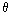AngPos(MS) degree
Angular speed: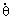AngSpeed(MS) degree/s
Angular acceleration: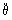AngAcc(MS) degree/s²

## Physical quantities

The list of physical quantities computed in the post-processing module and the names of the corresponding Flux parameters are given in the table below; in this table MS indicates the concerned mechanical set.

Torque Name Unit Explanation
Electromagnetic torque: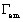TorqueElecMag(MS) N.m
Internal system
Friction torque: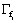TorqueFrInt(MS) N.m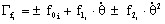Spring return torque: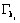TorqueSpInt(MS) N.m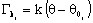Inertia torque: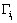TorqueInInt(MS) N.m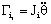Resistant torque*: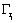TorqueReInt(MS) N.m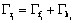or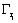External system
Friction torque: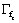TorqueFrExt(MS) N.m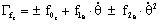Spring return torque: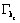TorqueSpExt(MS) N.m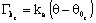Inertia torque: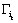TorqueInExt(MS) N.m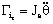Resistant torque *: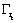TorqueReExt(MS) N.m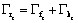or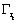Note: * The resistant torque is:
• either the sum of the friction torque + the spring return torque
• or the resistant torque defined using a formula

## Reminder of the solved mechanical equation

The solved mechanical equation for rotating motion is: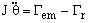where:

• J is the moment of inertia:•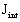is the moment of inertia of the moving mechanical set itself (internal system)
•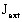is the moment of inertia of the external load (external system)
•is the electromagnetic torque
•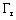is the resistant torque: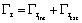•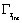is the resistant torque acting on the moving mechanical set (internal system)
•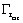is the resistant torque acting on the external load (external system)

## Important note

When there are symmetries or periodicities, the computed values of the torque correspond to the entire device.